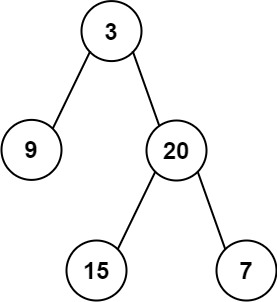# 106. construct binary tree from inorder and post order traversal

Given two integer arrays inorder and postorder where inorder is the inorder traversal of a binary tree and postorder is the postorder traversal of the same tree, construct and return the binary tree.

Example 1:Input: inorder = [9,3,15,20,7], postorder = [9,15,7,20,3] Output: [3,9,20,null,null,15,7]

• code
``````class Solution:
def buildTree(self, inorder, postorder):
if not inorder or not postorder:
return None

root = TreeNode(postorder[-1])
inorderIndex = inorder.index(postorder.pop())

root.right = self.buildTree(inorder[inorderIndex+1:], postorder)
root.left = self.buildTree(inorder[:inorderIndex], postorder)

return root

``````

must assign root.right at first, then root.left. Since in post order, when root pop out, from the right side, the next is the right tree.